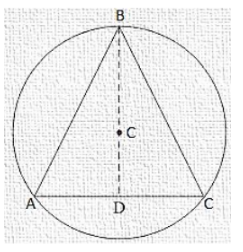# An equilateral triangle of side 9 cm is inscribed in a circle. Find the radius of the circle.`
Question:

An equilateral triangle of side 9 cm is inscribed in a circle. Find the radius of the circle.

Solution:

Let ABC be an equilateral triangle of side 9 cm and let AD is one of its median.

Let G be the centroid of ΔABC. Then AG: GD = 2 : 1

We know that in an equilateral triangle, centroid coincides with the circum centre.

Therefore, G is the centre of the circumference with circum radius GA.

Also G is the centre and GD is perpendicular to BC.

Therefore, In right triangle ADB, we have$A B^{2}=A D^{2}+D B^{2}$

$\Rightarrow 9^{2}=\mathrm{AD}^{2}+\mathrm{DB}^{2}$

$\Rightarrow A D=\sqrt{81-\frac{81}{4}}=\frac{9 \sqrt{3}}{2} \mathrm{~cm}$

$\therefore$ Radius $=\mathrm{AG}=\frac{2}{3} \mathrm{AD}=3 \sqrt{3} \mathrm{~cm}$